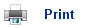# Solubility Products

• Nothing is insoluble; there will always be some substance dissolved in solution, no matter how small.
• The increase in entropy associated with dissolving ensures that this is so.
• Thus, even on a beach, rain water will leach out the silicon dioxide and transport it to the sea.
• A sparingly soluble ionic solid, such as calcium carbonate, in contact with a saturated solution of its ions is an example of the following equilibrium:

CaCO3(s) + aq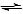Ca2+(aq) + CO32-(aq)

• As the solution is saturated, adding more solid will not push the position of equilibrium any further to the right; therefore the solid can be neglected from the expression for the equilibrium constant; this gives the following equilibrium constant:

Ksp = [Ca2+(aq)] [CO32-(aq)]

• This is known as the solubility product.
• There are solubility product values for all sparingly soluble solids, for example: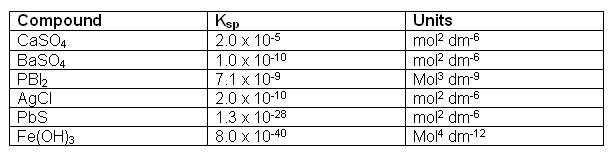• We can use the Ksp values to calculate the concentration of a particular ion within a saturated solution; for example, the concentration of chloride ions in a saturated solution of AgCl: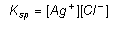• Equal amounts of Ag+ and Cl- must go into the solution, so: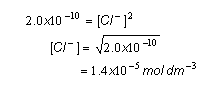• The Ksp value can also be used to determine the how other ions in the solution can affect the solubility of a sparingly soluble compound, for example, how adding extra Cl- or Ag+ ions will effect the concentrations.
• Imagine that we added some silver chloride to a 0.1 mol dm-3 solution of sodium chloride; the concentration of dissolved silver ions at equilibrium can be worked out.
• We have to assume that the all of the Cl- ions in the solution come from the sodium chloride (this can be done, as only a small amount of Cl- ions in the solution will come from the silver chloride). So therefore, the concentration of Cl- ions is 0.1 mol dm-3: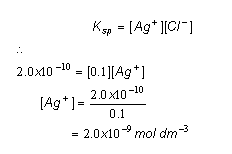## Useful books for revision

Revise A2 Chemistry for Salters (OCR A Level Chemistry B)Salters (OCR) Revise A2 ChemistryHome